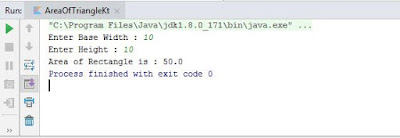# Program to find area of Triangle in Kotlin

Area of Triangle in Kotlin - This Kotlin program will read height and base of the Triangle to Calculate Area of the Triangle.

Source Code:
fun main(args: Array<String>)
{
print("Enter Base Width : ")
print("Enter Height : ")

val area: Double
if(base.toIntOrNull() != null && height.toIntOrNull() != null)
{
area = (base.toDouble() * height.toDouble()) / 2
print("Area of Rectangle is : \$area")
}
else
{
}
}
Output:
Enter Base Width: 10
Enter Height: 10
Area of Rectangle is: 50.0Kotlin program to find Area of Triangle - Output

Description:
In this program, we will take input from the user to enter to get base and height value of the triangle. To do so we have used readLine() function.

Next, we have checked that input accepted from the user is valid integer or decimal number. If an input is not valid then it will print the message to enter valid input.

To find the area of a triangle we have used an equation (base * height ) / 2, which is equivalent to (b*h)/ 2. Finally, an area of the triangle is printed using print() function.